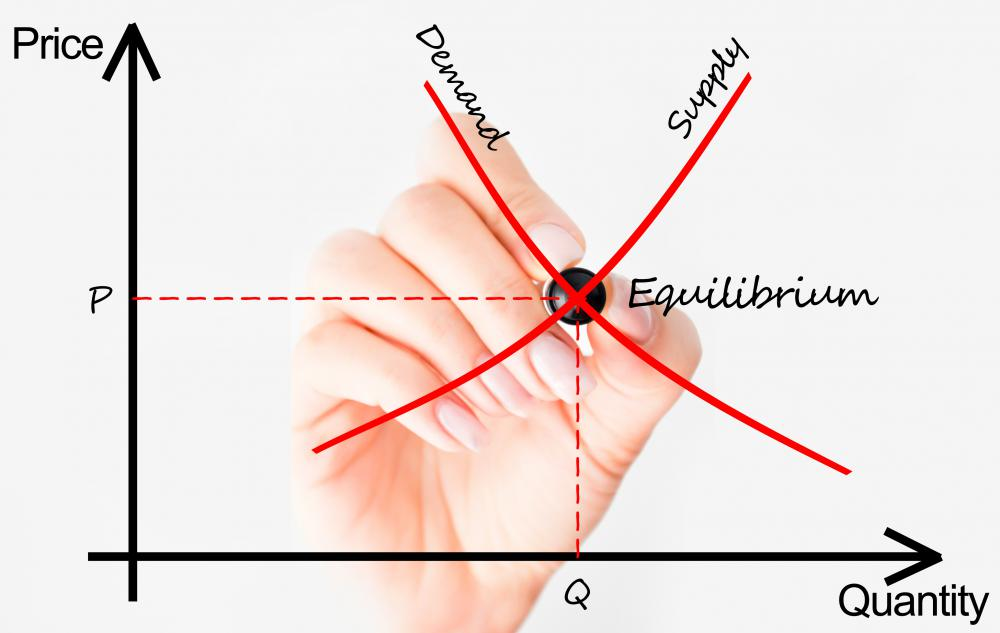# In Macroeconomics, what is Aggregate Demand?

Mike Howells

In macroeconomics, aggregate demand is a statistical measure that reflects the total demand present in a given economy at different levels of pricing. It is used both by itself and in conjunction with other measures, such as aggregate supply, in economic analysis. Alone, aggregate demand is also known as total spending, and can be used as a way of showing the total demand for a country's overall gross domestic product (GDP).When paired with aggregate supply, aggregate demand can be used to represent a supply and demand curve.

Aggregate demand can be calculated by adding together a country's total consumer spending, total capital investment by companies, total government spending, and the difference of its exports minus imports. The basic mathematical formula can be expressed like this, AD=C+I+G+(X-M). When calculated for different prices, an aggregate demand curve emerges, revealing lower levels of demand at higher prices, and increased demand at lower prices. On a chart that graphs price and quantity, this appears as a downward sloping straight line.

While the overall formula for this measurement appears relatively simplistic, each of the elements that must be added together can be complex in and of themselves. For instance, total consumer spending is actually comprised of consumer income minus taxes. Likewise, business investment usually depends on a number of factors, including the current interest rate. A higher interest rate means money is more expensive to borrow, which, in turn, means businesses will borrow and invest less.

Government spending, as it relates to aggregate demand, consists of everything from government worker salaries to money spent on tanks, agriculture, and welfare. It is typically one the largest single parts of the equation. The final part of it, exports minus imports, is generally referred to simply as net exports. This is heavily impacted by the exchange rate of a country's currency. A higher currency usually results in more imports and less exports, leading to an overall decrease in GDP.

When paired with aggregate supply, aggregate demand figures can be used to generate what is known as an AS-AD model. This appears on a graph with the demand as a downward sloping line, and supply as an upward sloping one, intersecting halfway. This point of intersection is known as the equilibrium point, and is the balance between price and production, where free markets tend to gravitate. This graph can be used to predict how various factors may impact a population's spending habits, among other things.

For instance, increasing unemployment would lead to less available income and, thus, a contraction in overall consumption. In turn this would move the aggregate demand curve to the left. The new equilibrium point would similarly shift left, lower down on the aggregate supply curve, to a new level of lower cost and lesser supply.

## You might also Like